français | español | polski | 日本語 |

Pokemon Math 24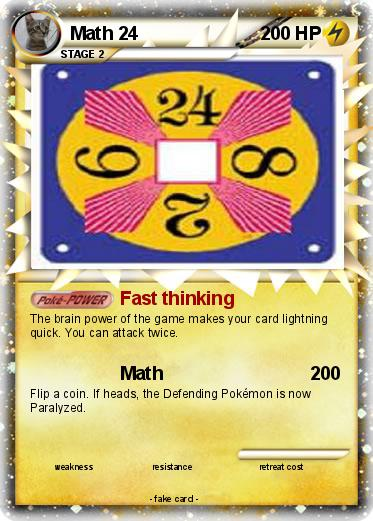Language Card : english

Creation Date : 13 November 2014

### Pokemon Passport

Name : Math 24

Serie : HeartGold & SoulSilver - Pokémon Prime

Type : Lighting

Attack 1 : Fast thinking
The brain power of the game makes your card lightning quick. You can attack twice.

Attack 2 : Math
Flip a coin. If heads, the Defending Pokémon is now Paralyzed.

### Vote for this card

(0 votes)

Related cards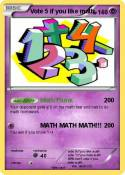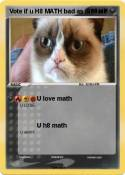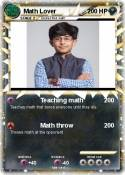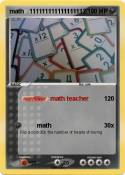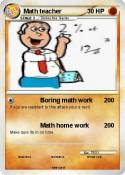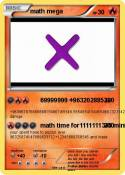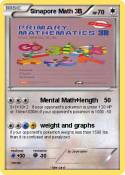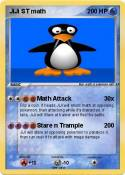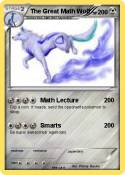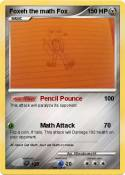Khan academy | Vote 5 if you hate math | Math Mann | I Can Do Math Everyday! | MATH | Math Teacher | MATh | math team | Math Book | Math knight | Brice | Math | Math Teacher | Math :( | math 100 x | me in math | Math man | Daniel EX | Nerd | math | Math Homework | Cool Math | math doesn't lie | Tushar | Math Knight | Math Man | Math | Boring Math Teacher | Math | Mrs Ferriola | 1+1 | Ratey hte math cat | St Math | Mrs Baker | baldi | Math | Math | Math | Math | more math | math | Asian guy | Asian guy | School | Math | Math Quiz | Vote if you like math | Matt's math degree | ??????????????? | njo | Math | Pi | ??? | math | PIE | can you Math????? | math pig | connor | math | M I C H A L | Homework | Mr pop | Nerdy | F-Man | = | Math | math | Bob | LEAFATARR | Kid

Comments

Pokémon is a registered trademark of Nintendo, Creatures, Game Freak and The Pokémon Company
MyPoKeCaRd.cOm 0.9 - Propulsed by jquery, php, gd and spip - XHTML 1 and CSS2 valid page
MyPokeCard.com is a funny site to design your own pokemon card, vote for the best pokemon cards and create pokemon colorings